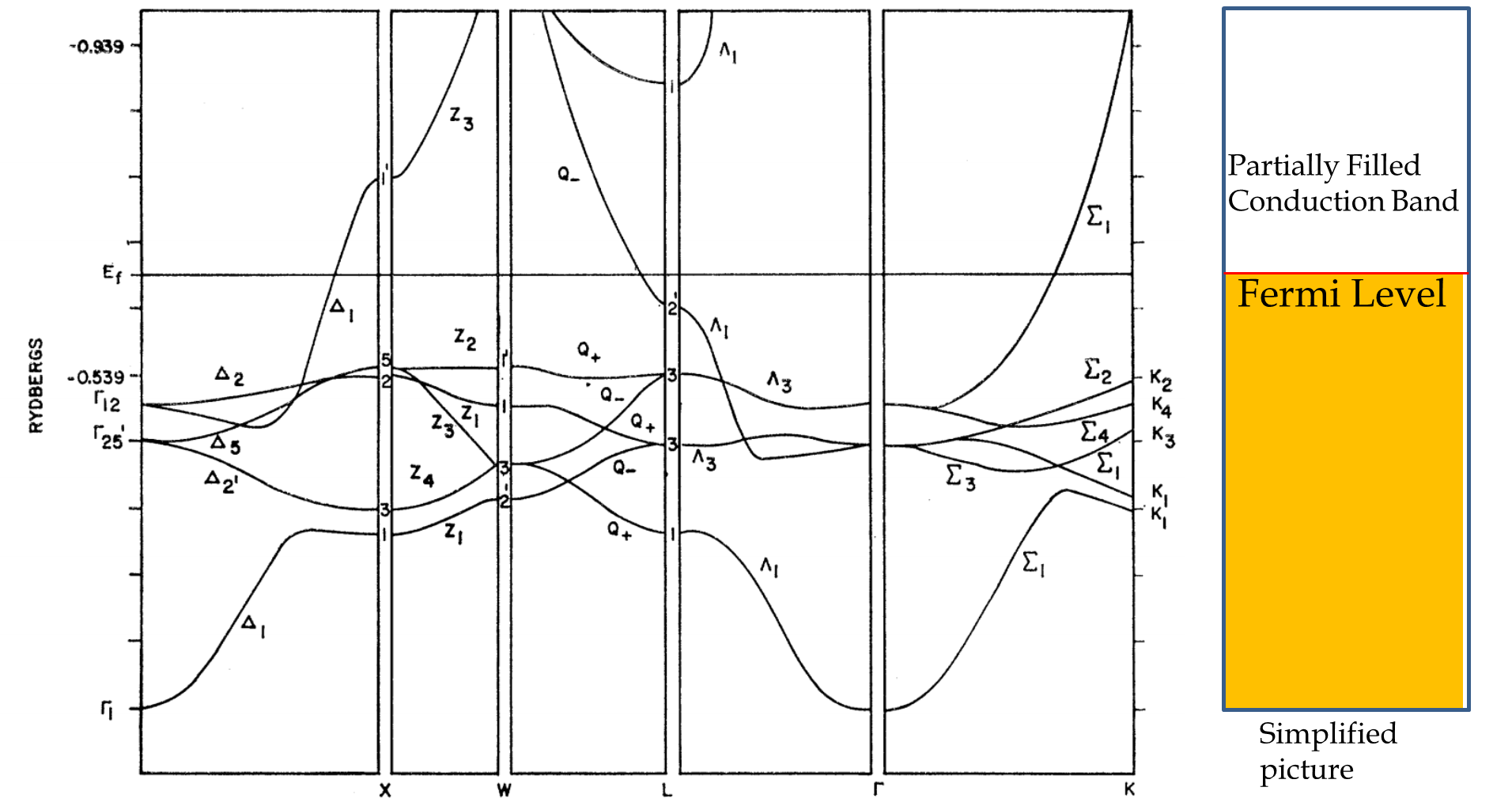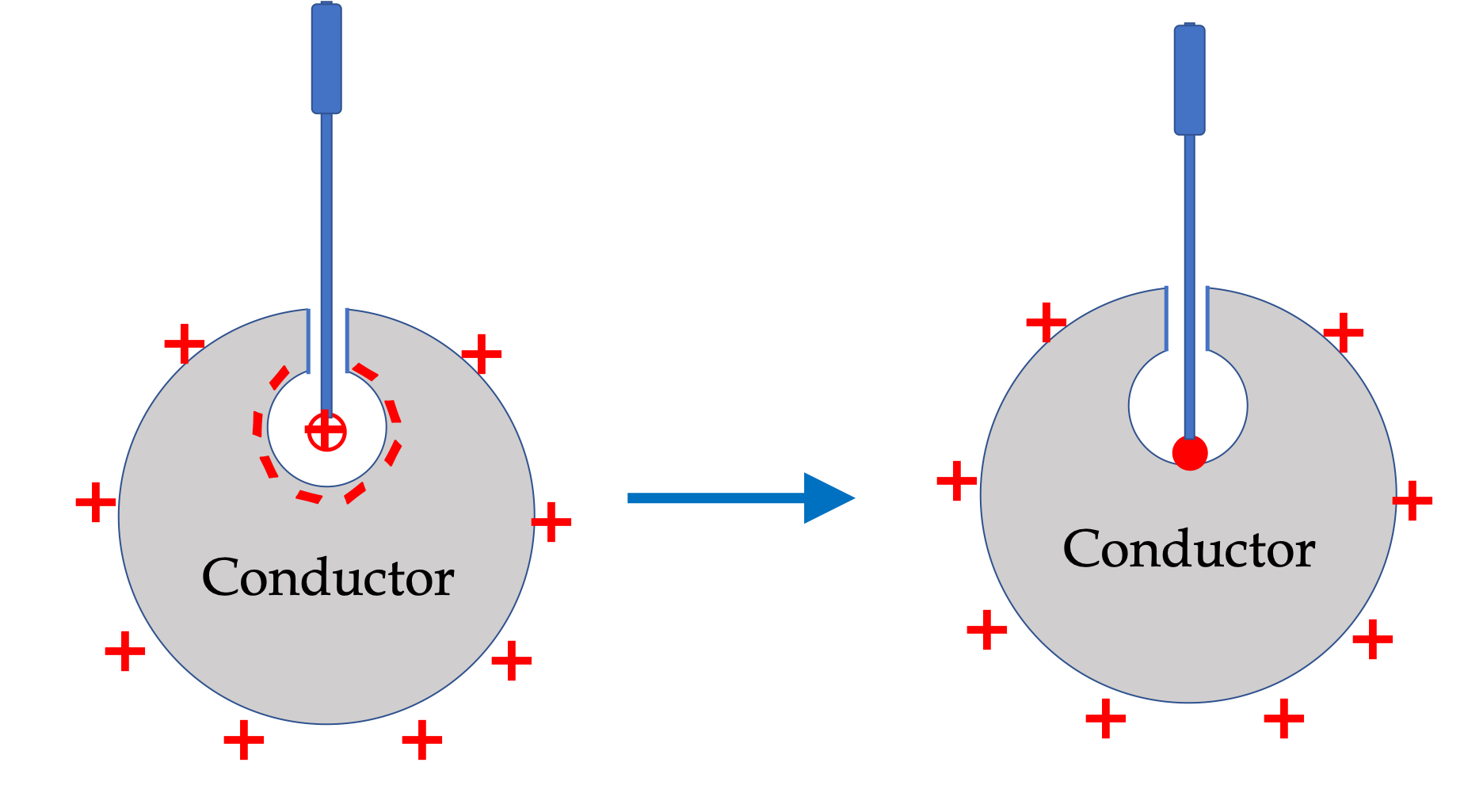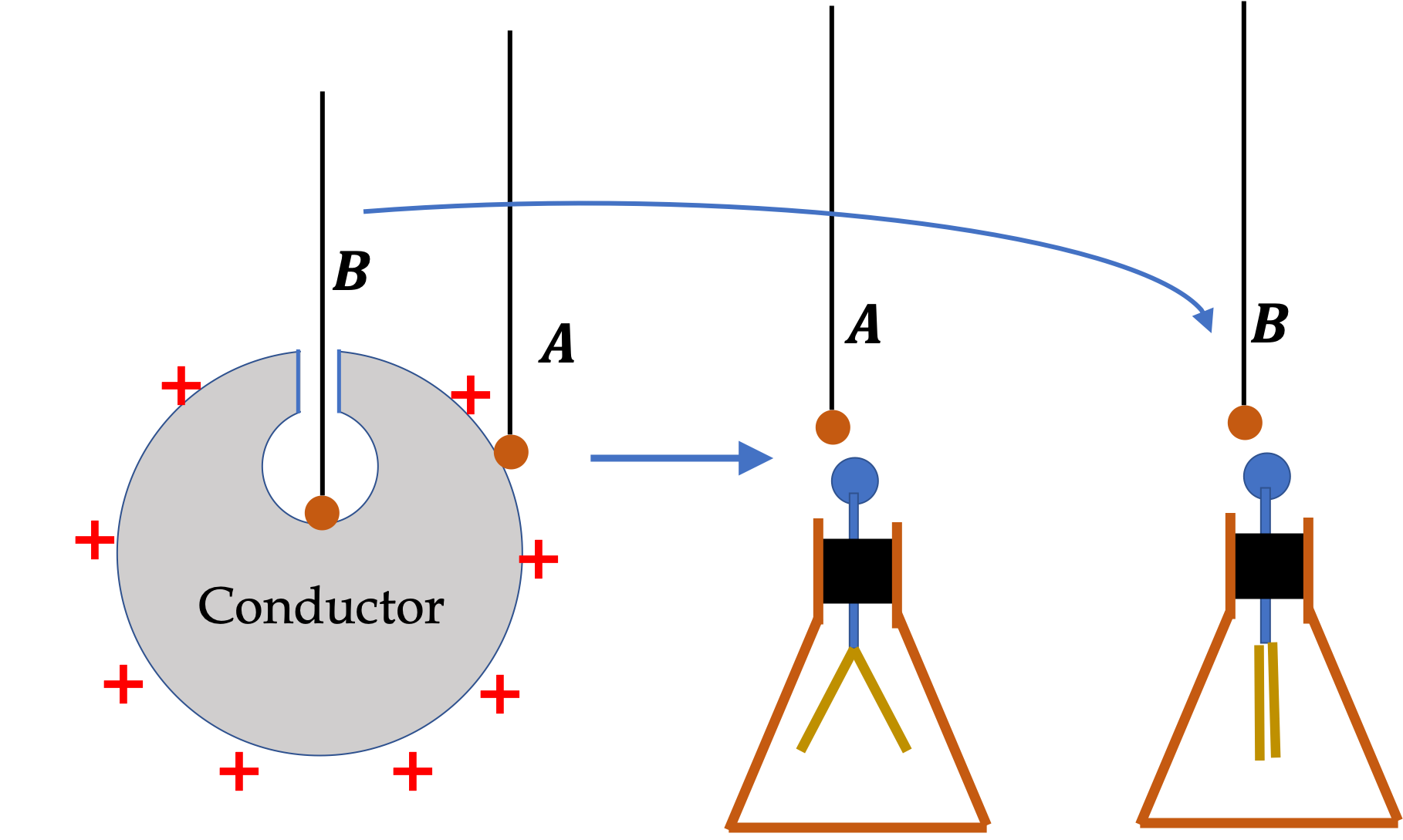## Section32.2Charged Metal

### Subsection32.2.1Conduction Electrons

Metals are good conductors of electricity. You need quantum mechanics to understand this behavior fully. We will just give bullet points here. Turns out that, in a metal, the highest energy band states of a metal, which is called conduction band, are only partially filled, leaving many states easily accessible without any jump in energy.

Electrons in the conduction band are called conduction electrons. Only a fraction of electrons in a metal are conduction electrons. For instance, in a piece of sodium, which is a good conductor, only one out of eleven electrons is condution electron. In copper, only one electron in 29 electrons is conduction electron.Figure 32.2.1. Energy bands of copper calculated by Glenn Burdick published in Physical Reviews, vol 129, p 138-150 (1963). Here $y$ axis has energy values and $x$ is wave vector magnitude and direction in the crystal, which is an indicator of the state. The simplified diagram summarizes the filling up of electrons in the states diagram, from lowest energy states to a final state, called the Fermi level, at zero degree Kelvin. You can see that states labeled $\Delta_1\text{,}$ $Q_2\text{,}$ and $\Sigma_1$ are only partially filled, meaning, states are continuously availble above the Fermi energy. In insulator, there will be a gap in energy before there are available states. Here, 1 Rydberg = 13.605 eV. This diagram is presented here only to illustrate a more complete picture of conduction you will study in a later course in physics.

### Subsection32.2.2Polarization of Metal Near an External Charge

When you place a piece of a metal near a positive charge, as shown in Figure 32.2.2 the conduction electrons in the metal will be attracted to the external positive charge and migrate freely towards that region.

The region the electrons move to now will have an excess of electrons over protons in the nuclei of atoms and the regions from where the electrons have migrated out will have more protons than electrons. Consequently, the metal will develop a negative region near the external positive charge and a positive region at the far end.

This separation of equal magnitude and opposite type is called polarization of the metal body. If you remove the external charge, the electrons migrate back and neutralize the positive region. The polarization of the metal happens only when there are external charges around.

You can think of polarization in terms of electric fields also as shown in Figure 32.2.3. The external charge creates an electric field, which we can call external electric field. For brevity, only three (blue) E-field lines from external charge are shown in the figure. Drift of conduction electrons leads to the polarization which creates electric field in addition to the external electric field. The external electric field and internal electric field cancel each other inside the metal when conduction electrons in the metal have stopped drifting.

\begin{equation*} \vec E_\text{net,in metal} = \vec E_{\text{external } q} + \vec E_{q\text{ on metal} } = 0. \end{equation*}

Separation of charges always causes the regions of extra charges on the metal on the outside surface as long as the external charge is outside the metal. This occurs even when there is a cavity in the metal. This is due to the fact that in the static equilibrium, electric field must be zero in the body of the metal. If metal has a cavity and external charge is inside the cavity, the inner surface of the cavity develops extra charge as illustrated next.

If an external charge is placed inside a metal cavity, as shown in Figure 32.2.5 metal is polarized with opposite charge at the surface of the metal inside the cavity and same charge as the external charge at the outisde surface. The total charge at the inner surface has the same magnitude as the external charge so that zero electric field flux through a surface with all surface points in the metal and surrounding the external charge and charges at the internal surface.

### Subsection32.2.3Charging a Metal

The inside surface versus the outside surface

An interesting property of a conductor in a static equilibrium is that extra charges on an isolated conductor end up at the outer surface of the conductor no matter where they originate from.

Consider a situationwhen we bring an external positive charge inside the cavity of a metal and then touch at the inside surface, as shown in Figure 32.2.6. Before, we touch metal with the external charge, inside surface the cavity is negatively charged and the outside surface of the conductor is positively charged. When we touch the inside surface of the cavity the induced charge is neutralized leaving the outside surface and the whole metal charged with a net positive charge.Figure 32.2.6. Illustration of what happens when you touch metal cavity. After we touch the inside cavity, the metal gains a net positive charge. Even when we touoched inside cavity, the end result will be a extra charges only on the outer surface of the metal.

### Subsection32.2.4Distribution of Charges on the Metal Surfaces

An electroscope is an ingenous device for detecting charges. It is based on two facts (1) charges flow in coductors and (2) like charges repel. In Figure 32.2.7, we illustrate the use of an electroscope to prove that there are no charges on the inside surface of the cavity.

Bring a small metal ball suspended by an insulating cord, touch the outside surface of the chaged metal, and then bring the ball near the knob of an electroscope. You will find that the leaves of the electroscope separate, proving that there is charge on the outside surface side of metal. Repeat the experiment by touching the metallic ball to the inside surface of the cavity. When you bring the metallic ball to an electroscope, now you will find no deflection, proving no charge on the inside surface.Figure 32.2.7. Electroscope shown on the right has two thin metal strips hanging by the metal rod. When a charge is brought near the metal knob at the top or touches the knob, the thin metals separate. You can use it to prove that inside surface of a metal cavity of an islolated charged metal is free of charge.

Another interesting aspect of charge distribution on a conductor is that charges tend to be denser where the curvature of the surface is greater as demonstrated by the charge distribution on an oblong-shaped metal. The surface charge density will be higher near the tip than the other side as shown in Figure 32.2.8.